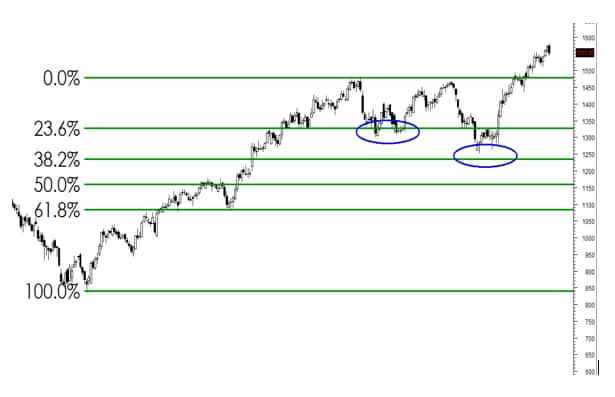# Classroom | How to interpret momentum indicators (Technical Analysis: Part 7)

## Relative Strength Index or RSI is a momentum indicator which measures the speed of price movement of the stock over a specified period of time.

November 27, 2019 / 09:48 PM ISTPart 7 of the Technical Analysis Classroom deals with reading momentum indicators to interpret the speed of price movement of stocks over a particular time period.

Q. What is the Relative Strength Index? What is its significance?

A. Relative Strength Index or RSI is a momentum indicator which measures the speed of price movement of the stock over a specified period of time. It measures the recent price changes to evaluate whether the stock is overbought or oversold.

RSI is plotted against the price chart and it moves between the values 0 to 100. A stock is considered to be overbought when the RSI is above 70 and it is considered oversold when RSI is below 30. The levels can be adjusted to better fit the security i.e. 80 and 20 or 90 and 10

Another important use of RSI apart from Overbought and Oversold is the RSI Divergence. If a stock price makes a new swing high or low, but at the same time RSI does not make a new high or low then it signals an RSI divergence. When a stock price makes a new high and RSI makes a lower high it is known as a bearish divergence and when a stock makes a new low but RSI makes a higher low it is known as a bullish divergence.

Close

Divergences are used to initiate contrarian trades.

Q. What is the Stochastic indicator? What does it indicate?

A. A Stochastic is a momentum indicator which shows the relative position of the recent closing price to the previous trading range over a specific period of time. It generates overbought and oversold signals for stocks and moves between the value 0 to 100

The current value of the stochastic indicator is referred as %K which is also known as a slow stochastic indicator and a 3-period moving average of %K is known as a fast stochastic indicator and is denoted by %D

It is considered a leading indicator as the indicator changes the direction before the stock price.

In an uptrend, the closing price tends to close near the upper end of the trading range and when the momentum starts to slow, the closing prices will start to retreat from the upper range causing the indicator to turn down at or before the final high of the security

Q. What are Bollinger bands? What is its significance?

A. Bollinger bands are set of lines that are plotted above and below a simple moving average of the stock price. The upper and lower bands are plotted two standard deviations away from the SMA line

Bollinger bands characterize the price and volatility of a stock. The bands automatically widen when the volatility increases and contract when volatility decreases.

A contraction in the band is a sign of low volatility and a possibility of volatility to increase in future and similarly wider bands have a possibility of volatility to decrease in future

Q. What is a Fibonacci series? How does one use this to identify trading opportunities?

A Fibonacci series is a series of numbers which starts with 0 and 1 and subsequent numbers are arrived at by adding the previous two numbers. So the series goes as 0,1,1,2,3,5,8,13,21,34 and so on. If you divide any number in the series with the previous number the ratio approximately comes at 1.618. Similarly, when you divide one number to the next number in the series the ratio approximately comes at 0.618 which is known as the golden ratio.

A similar consistency is found when any number in series is divided by a number which is 2 places higher or 3 places higher in the series. The ratio comes at 0.382 and 0.236 respectively.

It is believed that these ratios 61.8 percent, 38.2 percent, 23.6 percent finds its application in stock charts. Trading tools based on Fibonacci are Arcs, Fans, Retracements, Extensions and Time Zones. The most famous tool used is Fibonacci Retracements.

Q. What is a Fibonacci retracement? What does it indicate?

A. A Fibonacci retracement provides you with levels based on the Fibonacci series. It gives levels until where the price can retrace after the initial trending move in a stock price. An upward or a downward rally marks as the initial move or the trending move. Retracement is calculated from the peak or trough of such rally. After a sharp rally in a stock, it is prudent to wait for a retracement in prices. Such retracement can be up to Fibonacci levels of 61.8 percent, 38.2 percent, and 23.6 percent. For example, If the initial rally of the stock is of 100 points i.e. assume a stock has moved from Rs 500 to Rs 600, a 61.8 percent retracement suggests the stock retraces 61.8 percent of the Rs 100 up move i.e. Rs 61.8 and hence the stock price can retrace up to Rs 538.Likewise, the retracement levels can be 5.6 percent, 9.0 percent, 14.6 percent, 23.6 percent, 38.2 percent, and 61.8 percent.
Nooresh Merani
first published: Nov 27, 2019 09:48 pm